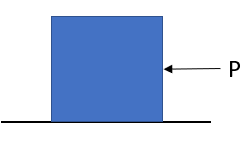## Newton's 2nd Law

A 5-kg block is sliding along a frictionless surface due to a constant force P = 20 N. At what time (in seconds) will the block be moving at 18 m/s?Hint
$$Force=mass\times acceleration$$$Hint 2 $$velocity=acceleration \times time$$$
Using Newton’s second law of motion:
$$Force=mass\times acceleration\rightarrow a=\frac{F}{m}$$$$$a=\frac{20N}{5kg}=4\frac{m}{s^{2}}$$$
Next,
$$velocity=acceleration \times time\rightarrow t=\frac{v}{a}$$$$$t=\frac{18m/s}{4m/s^2}=4.5\:seconds$$$
4.5 seconds